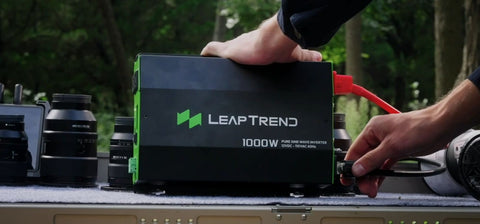# How Many Batteries Do I Need For a 1000 Watt Inverter?

It is crucial to understand the number of batteries required to power a 1000-watt inverter. This article will provide a detailed overview of how to determine the required number of batteries, including calculating the required capacity and providing examples. Read on to learn how to choose the appropriate number of batteries based on your requirements.

## 1. Assessing Battery Capacity

To determine the number of batteries required for a 1000-watt inverter, you need to consider the battery capacity. Battery capacity is typically measured in ampere-hours (Ah) and reflects the amount of current a battery can supply over a specific period.## 2. Calculating Battery Requirement

To estimate the number of batteries needed for a 1000-watt inverter, follow these steps:

Step 1: Determine the desired runtime. How long do you want the inverter to operate on battery power? Let's assume you want a runtime of 4 hours.

Step 2: Estimate the total power consumption. Multiply the inverter's power rating (in this case, 1000 watts) by the desired runtime. For example, 1000 watts * 4 hours = 4000 watt-hours.

Step 3: Take into account the battery's voltage. A 12-volt battery is commonly used with a power inverter 1000W.

Step 4: Calculate the required capacity in ampere-hours (Ah). Divide the estimated total power consumption in watt-hours by the battery voltage. In this case, 4000 watt-hours / 12 volts = approximately 333.33 Ah.

Step 5: Consider battery efficiency and take into account a safety margin. It's recommended to consider an efficiency factor of around 0.8 and add a safety margin of 20%. Adjust the required capacity accordingly. In this case, 333.33 Ah / 0.8 * 1.2 = approximately 500 Ah.

Step 6: Determine the battery capacity per battery. It depends on the available batteries in the market. For example, if you have access to 100 Ah batteries, divide the required capacity by the battery capacity per battery. In this case, 500 Ah / 100 Ah = 5 batteries.## 3. Example Scenario

Let's consider an example scenario to better understand the calculation:

If you want to power a 1000 watt power inverter continuously for 6 hours, using a 12-volt battery with an efficiency factor of 0.8, and assuming you have access to 150 Ah batteries, the calculation would be as follows:

Step 1: Desired runtime = 6 hours.

Step 2: Total power consumption = 1000 watts * 6 hours = 6000 watt-hours.

Step 3: Battery voltage = 12 volts.

Step 4: Required capacity = 6000 watt-hours / 12 volts = 500 Ah.

Step 5: Adjusted capacity = 500 Ah / 0.8 * 1.2 = 750 Ah.

Step 6: Battery capacity per battery = 150 Ah.

Number of batteries needed = 750 Ah / 150 Ah = 5 batteries.

In this example, you would require 5 batteries with a capacity of 150 Ah each to power the 1000W inverter continuously for 6 hours.

Determining the number of batteries needed for a 1000-watt inverter depends on the desired runtime, battery capacity, and efficiency. By following the calculation steps and considering battery efficiency and safety margins, you can estimate the required battery capacity and determine the number of batteries needed. Always consult the manufacturer's recommendations for battery compatibility and safety guidelines. With proper planning and consideration, you can ensure a sufficient power supply for your inverter.